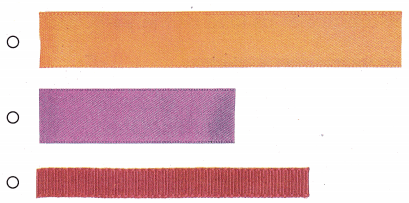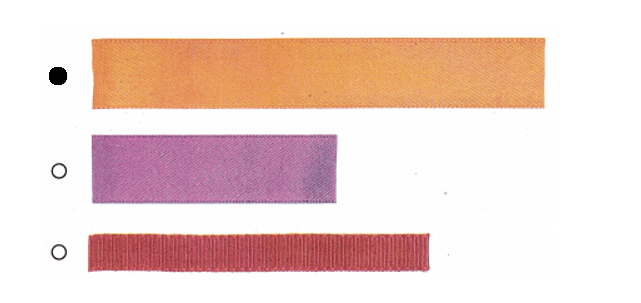Refer to our Texas Go Math Grade 1 Answer Key Pdf to score good marks in the exams. Test yourself by practicing the problems from Texas Go Math Grade 1 Lesson 17.1 Answer Key Length.

Explore

Use objects to show the problem. Draw to show your work.FOR THE TEACHER • Read the problem. Have I children use classroom objects to act it out. Rosa P has something that is longer than the drinking straw. She has another object that is shorter than the key. What objects might she have?
Longer than a straw Rosa P is having water bottle.
Shorter than a key Rosa P having eraser.

Explanation: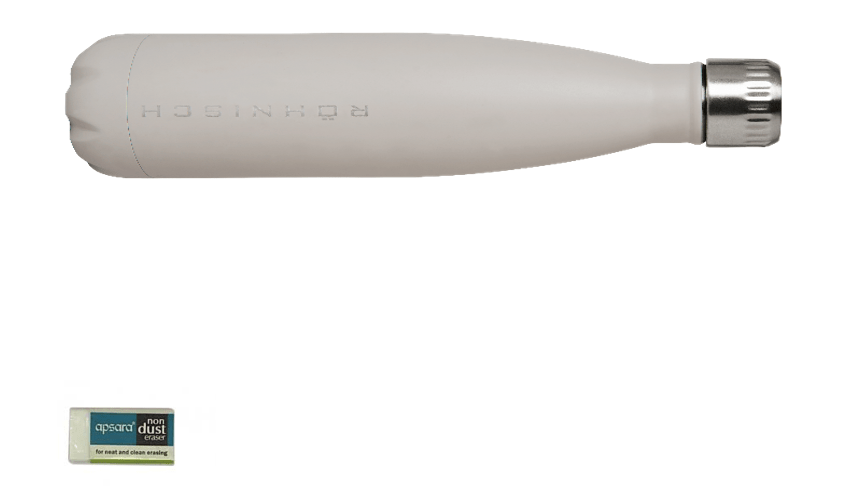Math Talk

Mathematical Processes
Compare the straw and the key. Which is longer? Which is shorter? Explain.
Comparison with length in the straw and a key:
Straw is longer than the key.
Key is shorter than the straw.

Explanation:
Comparison with length in the straw and a key:
Straw is longer than the key.
Key is shorter than the straw.Model and Draw
You can use string to measure the length of the pencil box.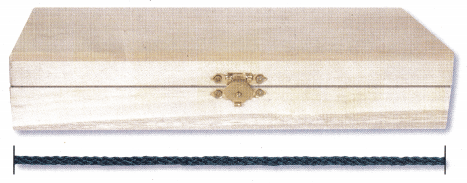The string is as long as the pencil box.
Objects longer than string will not fit.
The length of the pencil box is same as the string , so it can fit in the box.

Explanation:
Both string and box are of same length.
The string can fit in the box.

Share and Show
Use the string as a tool to measure the objects. Circle the objects that will fit in the pencil box.
Question 1.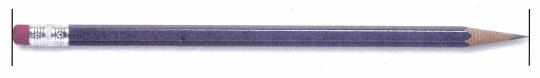Length of the pencil is more than the string length. So, pencil cannot fit in the pencil box.

Explanation:
Length of the pencil is longer than the length of the string.

Question 2.Length of the stick is less than the string length. So, it can fit in the pencil box.

Explanation:
Length of the stick is lesser than the length of the string.Question 3.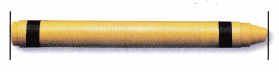Length of the crayon is less than the string length. So, it can fit in the pencil box.

Explanation:
Length of the crayon is lesser than the length of the string.Question 4.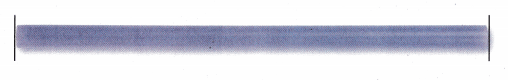Length of the plastic straw is same as the string length. So, it can fit in the pencil box.

Explanation:
Length of the plastic straw is same as the length of the string.Problem Solving
Use the string as a tool to measure the objects. Circle the objects that will fit in the pencil box.
Remember
The string is as long as the pencil box.

Question 5.Length of the plastic rod is less than the string length. So, it can fit in the pencil box.

Explanation:
Length of the plastic rod is less than the string length.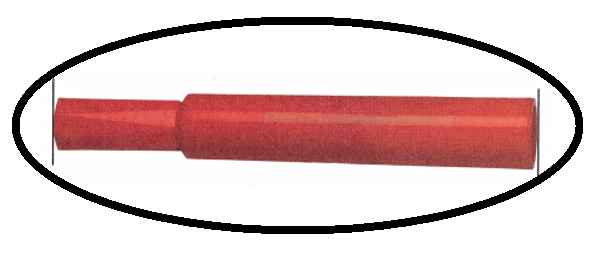Question 6.Length of the glue stick is less than the string length. So, it can fit in the pencil box.

Explanation:
Length of the glue stick is less than the string length.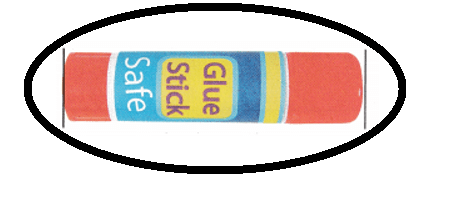Solve.
Question 7.
H.O.T. Multi-Step Barry is sending Jake his skateboard in a box. He used a ribbon to measure the skateboard. Explain how long the box needs to be?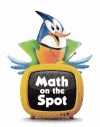Length of the box should be more than the skateboard length because it needs to fit in the box if not it cannot fit in the box.

Explanation:
Barry is sending Jake his skateboard in a box.
Length of the box should be more than the skateboard length because it needs to fit in the box if not it cannot fit in the box.

Question 8.
H.O.T. A box is as long as this ribbon. Find and draw an object that will fit in the box.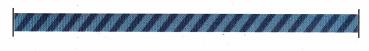Explain how you know the object will fit inside.
Crayon can fit in the box because its length is less than the ribbon/box.

Explanation:
Length of the crayon is less than length of the ribbon/box.Question 9.
Multi-Step A box is the same length as the ribbon. The ribbon is longer than the pencil. The ribbon is shorter than the straw and the spoon. Which object will fit in the box?
(A) straw
(B) pencil
(C) spoon
Explain why the other objects are not good choices.
Length of pencil is less than the length of the ribbon/box  it can fit where as spoon and straw lengths are more than ribbon/ box they cannot fit.
(B) pencil.

Explanation:
A box is the same length as the ribbon.
=> Length of box = length of ribbon.
The ribbon is longer than the pencil.
=> Length of ribbon > length of pencil.
The ribbon is shorter than the straw and the spoon.
=> Length of ribbon < Length of straw and spoon.

Question 10.
Texas Test Prep A box is the same length as this string. Use string to find which object will fit in the box.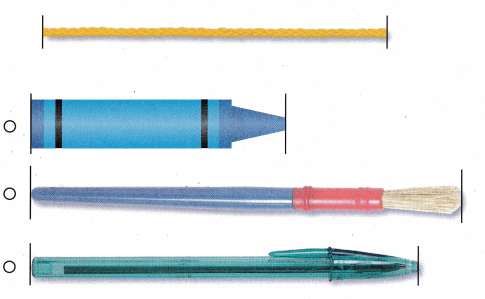Length of crayon and pen are less than the string length, they fit in the box not paint brush.Explanation:
Length of the crayon is less than the length of the string.
Length of the paint brush is more than the length of the string.
Length of the pen is less than the length of the string.

TAKE HOME ACTIVITY • Have your child explain to you how he or she uses o measuring tool to measure an object
Yes, my kids said they use the ruler to measure the objects.

Explanation:
Ruler is used by my kids for measuring objects.

### Texas Go Math Grade 1 Lesson 17.1 Homework and Practice Answer Key

Use string this long as a tool to measure the objects.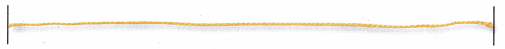Circle the objects that are longer than the string.

Question 1.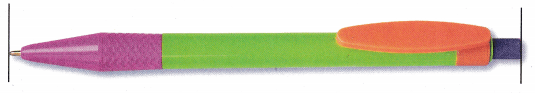Explanation:
Length of the pen is more than the length of the string.

Question 2.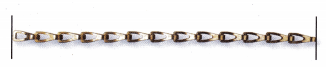Length of the chain is less than the length of the string.Explanation:
Length of the chain is less than the length of the string.

Question 3.Length of the strip is same as the length of the string.Explanation:
Length of the strip is same as the length of the string.

Problem Solving
Question 4.
Ava wants to make a bracelet as long as this piece of yarn. Draw a bracelet as long as the yarn.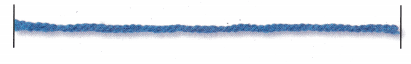Explain how you know the bracelet and yarn are the same length.Explanation:
Length of the bracelet is same as the length of the piece of yarn because measure them by placing starting point of them parallelly. If they both are same, their ending points will coincide if not smaller or longer they will be.

Lesson Check
Question 5.
A pencil case is the same length as this string. Use string to find which pencil will fit in the box.Length of the maroon pencil is less than the length of the string. So, it can fit in the box.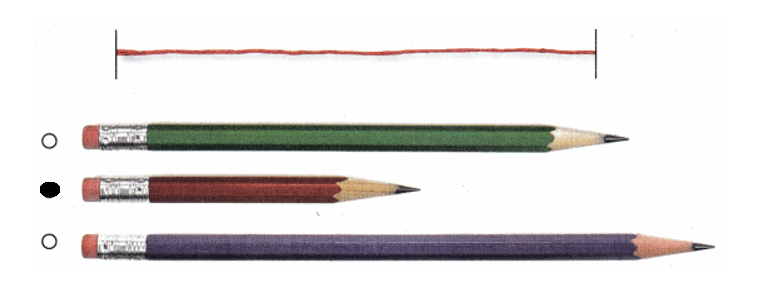Explanation:
Length of the green pencil is more than the length of the string.
Length of the maroon pencil is less than the length of the string.
Length of the violet pencil is more than the length of the string.

Question 6.
Elena wants to put objects inside this box.Which length of ribbon will be the best measuring tool to measure the length of the objects?